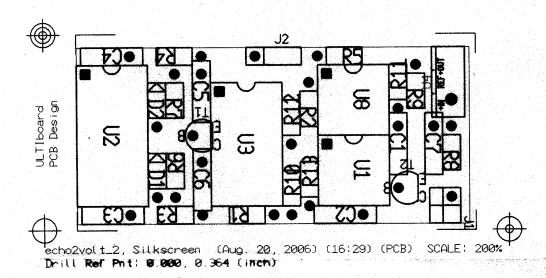# Distance Sensor PCB SilkScreen. Final version 20 aug 2006Konstruiert und fotografiert von Richard R. Budding. Hochgeladen am 21.8.2006, 17:41 von rbudding.  14 / 32

This is the component layout, checkout the number of components versus the amount of space within the 9v box….

wahsager (22.8.2006, 11:14:34)

Do you have a part list of this schematics?

rbudding (22.8.2006, 14:24:32)

Yes I have:

—- List of Components —-

``````    Name | Alias        | Shape      Comments

C1 = 100N         : (CRM5A)
C2 = 100N         : (CRM5A)
C3 = 470P         : (CRM5A)
C4 = 1N           : (CRM5A)
C5 = 100N         : (CRM5A)
C6 = 100N         : (CRM5A)
C7 = 2N2          : (CRM5A)
D1 = 1N4148       : (DIOD1)
D2 = 1N4148       : (DIOD1)
J1 = HDR_4        : (SIL4!0)
J2 = HDR_3        : (SIL3)
R1 = 220K         : (RES10)
R2 = 68K          : (RES10)
R3 = 6K8          : (RES10)
R4 = 100K         : (RES10)
R5 = 47K          : (RES10)
R6 = 47K          : (RES10)
R7 = 47K          : (RES10)
R8 = 68K          : (RES10)
R9 = 180K         : (RES10)
R10 = 47K          : (RES10)
R11 = 390K         : (RES10)
R12 = 47K          : (RES10)
R13 = 100          : (RES10)
T1 = BC550        : (TRA_TO92B)
T2 = BC560        : (TRA_TO92B)
U1 = NE555        : (DIP8S)
U2 = 4528B        : (DIP16S)
U3 = 4016         : (DIP14S!0)
U4 = 7805         : (78MXXV)
U8 = CA3240       : (DIP8S)``````

rbudding (25.8.2006, 14:37:24)

as you can see the items are not expensive! Pretty standard material.

rbudding (28.8.2006, 16:11:44)

items have been ordered today, prints will be delivered next week, I ordered 50 last friday.

….

Richard